[Home] [Science]

# The Equation of Time

### and indeed, the motions of the Earth about the Sun more generally

Against the backdrop of the distant stars, the Earth has two simple and regular motions. It turns on its axis once every 23 hours, 56 minutes, and 4.1005 seconds, and it orbits the Sun once every 365 days, 6 hours, 9 minutes, and 9.7676 seconds (these figures being those which applied at the start of the year 2000).

The usual day that we're used to, of course, is 24 hours in length, and the usual year that we're used to is slightly less than 365 days and 6 hours, since in the calendar, the leap year day in one out of every four years is omitted three times in every 400 years.

The latter discrepancy is due to the precession of the equinoxes; the natural year of the seasons, relative to the direction the Earth's axis is pointing, differs from the sidereal year because that direction slowly changes. Thus, the tropical year is 365 days, 5 hours, 48 minutes, and 5.1875 seconds.

The former discrepancy is one that confuses many people when they see introductory books about astronomy.

After all, it's because the Earth turns on its axis that we have day and night. Our clocks are made to indicate the same time of day after 24 hours. So if the Earth didn't turn to bring the place where one lives to face the Sun in 24 hours, but after a shorter period of time, those extra four minutes each day would add up.

If you do add them up, though, you can see what is going on. Four minutes a day add up to an hour in 15 days, so they add up to two hours in a month, or 24 hours in a year.

So, if our clocks were wrong by four minutes a day, in six months we would be having lunch at the middle of the night!

And that's a clue to what's going on. In six months, since the Earth goes around the Sun once a year, we will be on the opposite side of the Sun. If we don't think of the Earth's rotation in terms of rotation relative to which direction the sun is in, but just its rotation by itself, then we get a period of rotation that is different, by four minutes a day, from the regular clock day of 24 hours.

The 23 hour, 56 minute, and 4.09 second axial rotation period is almost exactly uniform; seasonal wind patterns actually affect the Earth's rotation to an extent now detectable with today's atomic clocks; this is known as the UT1 - UT2 correction.

The 24 hour day that we normally use to regulate our lives by is uniform too, but that's because we get it from clocks. Of course, that doesn't count those two occasions on the weekend when we "spring ahead" and "fall behind", to adjust those clocks for Daylight Saving Time. But the actual relationship of locations on the Earth to the direction of the Sun is more complicated, and so the time indicated by a sundial differs by clock time in a pattern over the course of a year known as the Equation of Time.

To be complete, we might also consider the anomalistic year; the position of the Earth's aphelion also shifts very slowly, leading to the period between two consecutive times at which the Earth is farthest from the Sun being 365 days, 6 hours, 13 minutes, and 52.53865 seconds. When calculating the Earth's position using Kepler's Equation, it is the time within this year that is used as the starting point, to obtain a variable known as the mean anomaly.

Given the figures above, in 24 hours, the Earth turns on its axis by 360.98560556 degrees, and, on the average, the Earth moves 0.98560927 degrees in its orbit around the Sun. If you subtract the second figure from the first, you get 359.99999629 rather than exactly 360 degrees; this corresponds to the fact that we currently use, for timekeeping, a second whose length is based on astronomical observations made from the years 1750 to 1892, and the Earth's rotation is gradually being slowed by the tides. (Since the references I could find simply had the day about one millisecond too long in the year 2000, by averaging out the bumps in a graph of the difference between mean solar time and atomic times, I took the Earth as losing 10.6 seconds in every 20 years at that time, which makes the day 1.45 milliseconds too long, and used that as my starting point in calculating the sidereal sidereal day, as opposed to the more commonly-seen tropical sidereal day.)

At this moment, of course, it is now both where you are and everywhere else on Earth. But the time that is now is still called by different names in different places.

Because the Earth is round,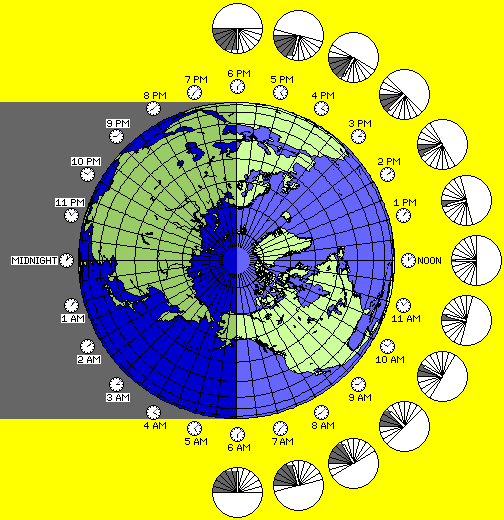while the actual time is the same everywhere, the time of day is not; one part of the world is under the rays of the noonday Sun, and another is beneath the starry skies of night.

This picture represents the Earth as it would be at 4:07 PM UTC, or 12:07 PM Atlantic Standard Time, on March 21, the date of the Vernal Equinox.

Thus, the division between night and day is shown as corresponding to a great-circle pair of meridians of longitude; over the central meridian for Atlantic Standard Time, 60° West longitude, the sun's rays fall to the Earth vertically on the Equator, and elsewhere on that meridian, the solar time is also exactly noon. On that date, though, the clock time happens to be running seven minutes later than the solar time, due to the Equation of Time which we will be discussing here.

So, the Sun establishes, by means of the angle between the line from a spot on the Earth to the Earth's center, as projected to the plane of the Equator, and the line from the Earth to the Sun, a framework that gives the time of day everywhere on the Earth.

A simple way to understand why the Earth's axial rotation period is only 23 hours, 56 minutes, and some 4 seconds when the day is 24 hours long is to think of the Earth, in its rotation, as being like the hour hand of a 24 hour clock,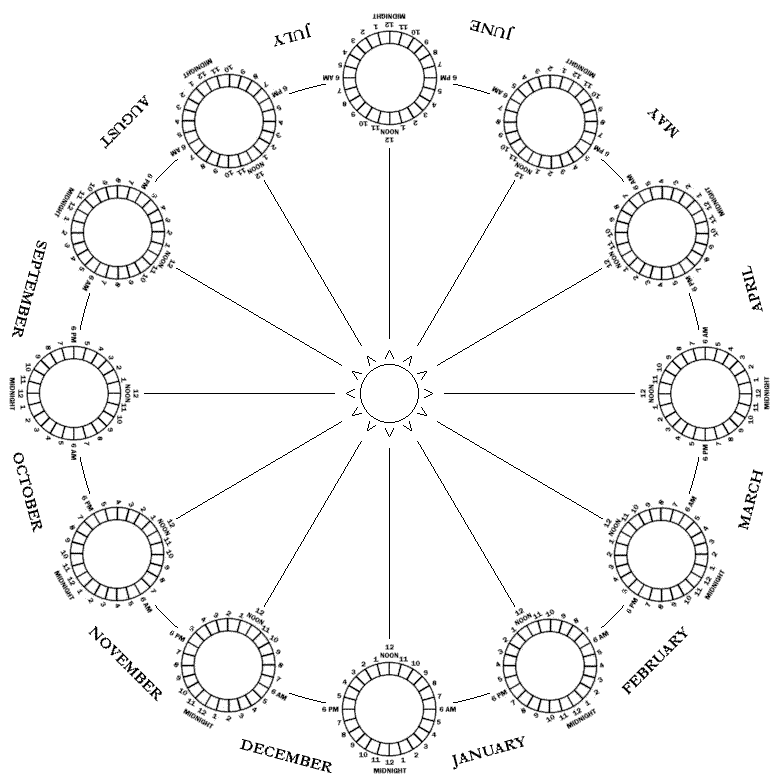which has to move fast, because the dial is also rotating slowly, moving two hours ahead every month.

The hour hand is the line between any given location and the center of the Earth, and the dial is the framework of local times within which the Earth rotates established by the light coming from the Sun.

And, of course, both the dial and the hour hand are rotating counter-clockwise.

The 23 hour, 56 minute, and 4 second axial rotation period is also sometimes referred to as the sidereal day, even though it doesn't represent the amount of time between day and night. This is because, for any one evening of astronomical observation, the annual motion of the Earth can almost be neglected; the stars in the sky appear to move around an observer once in the course of a day, just as the Sun appears to do. This meant that it was convenient, in drawing maps of the stars in the sky, to express the coordinate known as Right Ascension, which corresponds to longitude in a map of the Earth, in terms of hours and minutes instead of degrees. Since the 23 hour, 56 minute, and 4 second period is the time required for the stars to move through 24 hours worth of Right Ascension, it seemed natural to refer to the Earth's axial rotation period as a sidereal day, even if the term has some potential to be confusing.

The Equation of Time, which gives the discrepancy between the time as we see it from clocks (exclusive, of course, of our doing such strange things with them as resetting them for what is variously known as Daylight Savings Time in North America or Summer Time in the United Kingdom, and of the correction required because we may not live precisely on the central meridian of our Standard Time Zone) and that on a sundial indicates a discrepancy which can be as much as a quarter of an hour at some times of the year.

Given, as noted above, that the Earth turns regularly on its axis, to a very high degree of precision, as indicated by transit circle observations of stars passing overhead (very minor discrepancies can now be detected by today's very accurate atomic clocks), the only way we can account for this is that, in the course of the year, the Sun is not quite in the direction from the Earth that we might expect on the assumption that the Earth's orbit around the Sun is a perfect circle (plus one other hidden assumption that I will mention later).

By Kepler's laws, the Earth's orbit around the Sun is an ellipse. It is an ellipse that is very nearly a perfect circle; only the planets Venus and Uranus have less eccentric orbits than that of the Earth. Also, the Earth's motion sweeps out equal areas in equal times within that ellipse by Kepler's laws.

The effect of that on the position of the Sun in the sky would be the following: on or about July 4th, when the Earth is the farthest from the Sun, and six months later, when the Earth is the closest to the Sun, a sundial would give the correct time. In the fall, the Earth would not have moved in its orbit around the Sun from aphelion by the angle that one might expect, and so the apparent solar days will have been shorter, being more sidereal-like, and the sundial would run ahead; and in the spring, the sundial would be late.

The diagram below illustrates this phenomenon: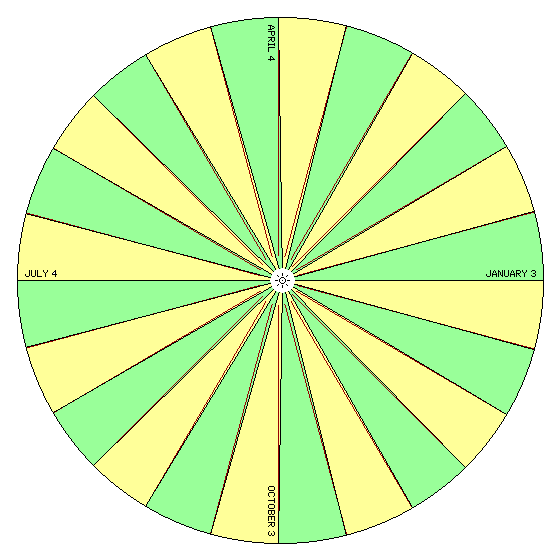it is drawn to scale. The Earth's orbit appears to be a circle, not an ellipse, in the diagram, because the extent to which it is flattened is only about one part in 7,000, which is too small to show on this scale. But the ratio of perihelion to aphelion distances for an orbit of the eccentricity of that of the Earth is about as 261 to 265, and this, the extent to which the Sun is not at the center of the orbit, can be shown. And, because of that, the effect of the law that equal areas are swept out in equal times can also be shown to scale on the diagram.

Thus, the orbit is divided into twelve segments of equal duration, and the areas they sweep out are colored alternately green and yellow; the dark red lines within those colored areas indicate the direction in which the Sun would be expected to lie if the Earth moved uniformly in a circular orbit with the Sun at the center.

Incidentally, it may be noted that the lines showing where the Sun might be expected to be found, which had to be drawn quite long so that it was clear how they differed from the lines from the Earth to the Sun, all seem to be pointing to a single point to the left of the Sun in the diagram.

There is a reason for that.

As noted, in shape, the difference between the Earth's orbit and a circle is very small, but the distance of the Sun from the center of the orbit was the noticeable result of the orbit being an ellipse. In such a case,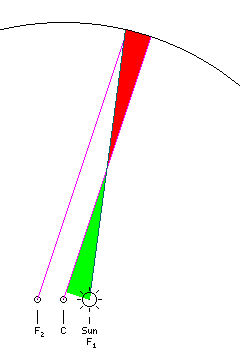if I wish to draw a line from the Sun enclosing a certain fraction of the area between that line and the line of perihelion, one way to do it is to draw a line first from the center of the circle enclosing that fraction of its area.

Then, if I surrender the area given by the green triangle, and take instead the area given by the red triangle, by drawing a line from the Sun to the circle of the orbit which crosses the line from the center enclosing the fraction of the area desired, since the two triangles are about equal in area, I closely approximate the line to the Earth's actual position.

The line from the center of the circle, since it enclosed the desired fraction of the circle, has a slope representing the fraction of the year that has passed from perihelion; it moves at a uniform angular speed. If one takes a line in a similar direction from the Earth, it then will point to a point as far to the left of the center as the Sun is to the right of the center, or to the other focus of its elliptical orbit.

So the elliptical motion of the Earth around the Sun is approximated by movement in a circle around a center halfway between the two foci of the ellipse which is uniform in angle as viewed from the other focus of the ellipse. This approximation, with the other focus of the ellipse called the equant, is how Ptolemy described the apparent annual motion of the Sun, and how the elliptical orbits of the planets, turned into scaled circular orbits around the Earth, or deferents, were also described, an epicycle then giving the displacement due to the fact that the other planets, and the Earth, actually orbit the Sun.

Kepler's Laws, with the ellipse being determined by the Sun at a focus, and with areas swept about by a line from the planet to the Sun, base orbital motions on the Sun directly; this approximation seems to give two imaginary points, the center of the orbit, and the other focus of the ellipse, the primary role in determining a body's motions, and thus many histories of astronomy have denounced the ancients for settling for something so unaesthetic. However, without such evidence as Tycho Brahe's and Kepler's accurate observations of Mars, it is unclear how one would have expected them to do better purely through artistic imagination, even if the boldness of Einstein seems to provide the example.

But the actual Equation of Time, as one can see it graphed in many references, has two large bumps and two smaller ones in the course of a year. So there must be something else going on.

The only other thing about the Earth's orbit that comes to mind is that the Earth's axis is tilted by about 23.44 degrees, relative to its orbit around the Sun. We all know how that causes the seasons. But what would that have to do with the time of day?

If you look at a diagram of the Earth's seasons, such as you might find in many atlases, you might indeed wonder. The Earth is usually pictured in such a diagram at the two solstices and the two equinoxes, and it is apparent that at these four times of the year, the Sun would cross one's meridian in the sky at precisely noon local mean solar time if the Earth's orbit around the Sun were circular.

One has to look at the effects of the Earth's tilt a bit differently to see that, yes, indeed it does affect the relationship of the Earth to the Sun in such a way as to shift the time we would see on a sundial.

If we looked down on the Earth and the Sun from the vantage point of the star Polaris, then, had the Earth's orbit around the Sun been a perfect circle, what we would see instead (through a powerful telescope!) would be a foreshortened ellipse with the Sun at the center.

The difference between these two views of the Earth's orbit is illustrated by the diagram below, which shows a representation of the Earth's tilt causing the seasons in a conventional form, and then the same diagram so that the Earth's axis is shown as vertical, and the Ecliptic plane is tilted, as better illustrates the Equation of Time: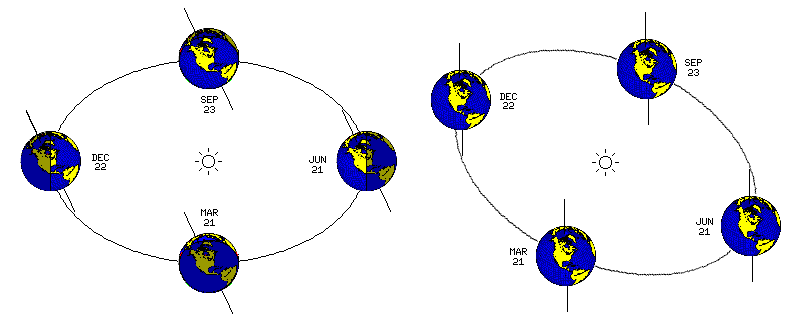The Earth would be closest to the Sun at the two solstices, when it is highest and lowest relative to the Sun in the Earth's equatorial plane, and farthest from the Sun at the two equinoxes. Between these four phenomena, the year is divided into four even quarters, and the angle at which the Earth is seen from the Sun differs by exactly 90 degrees from one of them to the next.

But because it is a flattened ellipse, halfway between one solstice and the following equinox, or one equinox and the following solstice, instead of having a nice 45 degree angle, the Earth is closer to its equinoctial position than one might expect, as illustrated by the diagram below:This leads to a discrepancy where the sundial is ahead twice a year, and behind twice a year, and in both cases by equal amounts. (Incidentally, the diagram above is drawn to scale, and, thus, as the angular discrepancy between the actual direction of the Sun, and the direction in which we would expect to find it is readily visible in the diagram, the error in the time on a sundial due to this cause is also genuinely noticeable.)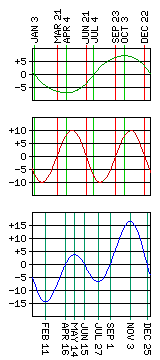The combination of this discrepancy and the one due to the Earth's elliptical orbit and Kepler's Laws gives the characteristic form to the Equation of Time with which we are familiar, as shown in the diagram to the right.

The dark green curve in the top graph shows the effect due to the Earth's orbit being elliptical. The vertical green lines that cross it show the days of perihelion and aphelion, when this effect is zero, and the days halfway between them.

The dark red curve in the middle graph shows the effect due to the tilting of the Earth's axis. The vertical red lines that cross it give the dates of the equinoxes and solstices, when this effect is zero.

The blue curve in the bottom graph shows the actual Equation of Time, resulting from the combination of these effects. It is crossed by vertical dark blue-green lines, giving the dates when the sundial and the clock coincide and the greater and lesser maximae and minimae of this equation.

Looking at this graph at the right, we now see why there had to be the slight extra complication in the first diagram on this page of having all the clocks show a time seven minutes after the hour.

After all, it shows the dividing line between night and day exactly crossing the Earth's poles. This means that it illustrates the Earth at one of the equinoxes. If it were at the vernal equinox, at about March 21, then instead of pointing straight up, the minute hands of all the little clocks in the diagrams ought to be pointing to about seven minutes after the hour, because on that date, the sundial is about seven minutes slow compared to the clock. If it were at the autumnal equinox, at about September 23, from the graph you can see that the sundial is running seven minutes fast, so the minute hands all ought to be at seven minutes before the hour.

[Home] [Science]